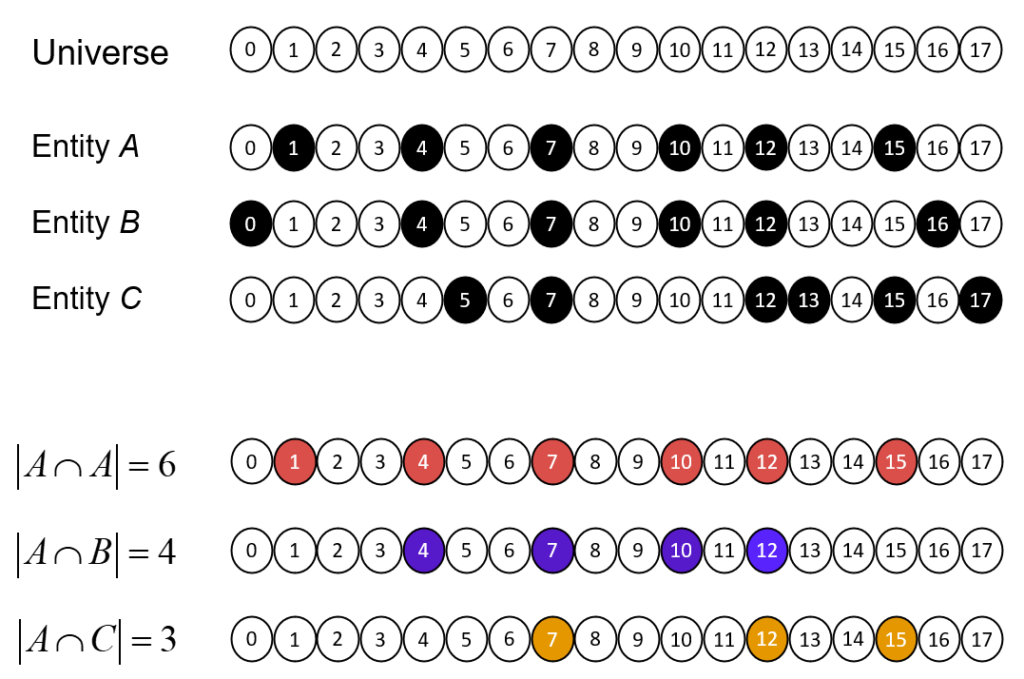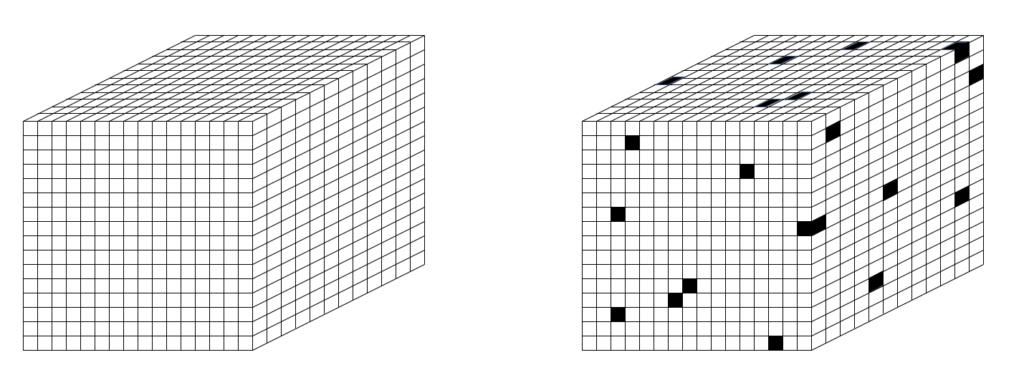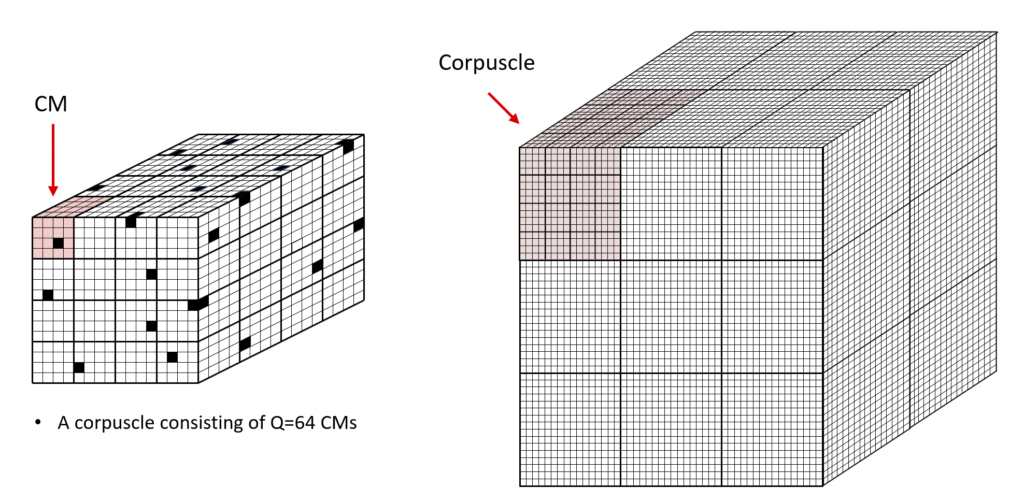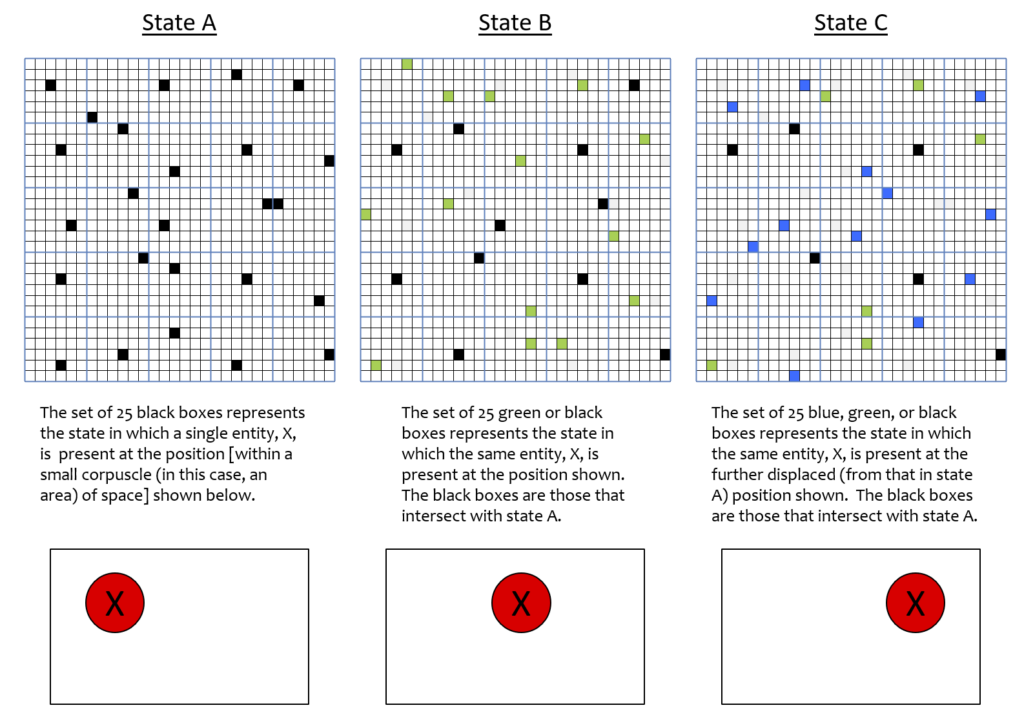# A Physical Theory based on Sets, Not Vectors: Part 1

In quantum theory (QT), physical states are formally represented as vectors (in Hilbert space). As we all know, a vector is equivalent to a point, which is 0-dimensional, and therefore has zero extension. This suggests that any physical state, and thus any physical particle that is part of the ensemble present in that state, formally has zero extension. However, quantum theory avoids this interpretation by making the Hilbert space dimensions themselves, i.e., its basis vectors, be functions of space, not simply scalar-valued. Specifically, the value of any particular dimension is the absolute value of a wave function, i.e., of a particular probability density function over space. This is the mechanism by which quantum theory imparts spatial extent to physical entities. It is why quantum theorists have been forced to say that the formal mathematical objects, i.e., the probability density functions, used to represent physical objects are more real than the physical objects themselves. And, it’s the underlying reason why in QT, all states that can exist actually do simultaneously exist, and furthermore, simultaneously exist at all instants of time. Somehow, they all occupy the same physical space, i.e., they all exist in physical superposition, and they do so for all of time. Make no mistake: quantum theory says that at every moment of time, all possible physical states exist in their entireties: in particular, QT does NOT say that any particular state partially exists. Rather, it says that the probability of actually observing any state, all of which fully physically exists, is what varies.

The choice to represent states as vectors can perhaps be considered the most fundamental assumption of QT. It implies that the space in which things arise and events occur exists prior to any of those things or events. This seems a perfectly reasonable, even unassailable assumption: indeed, how could it be otherwise? How can anything exist or anything happen unless there is first a space (and a time) to contain them? But that assumption is assailable. In fact, there is a simple formalism that completely averts the need for a prior space to exist. That formalism is sets. We can build dimensions, and therefore a space, out of sets. Specifically, a formal representation of dimension can be built out of, or emerge from, a pattern of intersections amongst sets, specifically amongst subsets chosen from a universe of elements, as explained in Fig. 1.

At top of Fig. 1, we show a universe of 18 binary elements. These elements happen to be arranged in a line, i.e., in one dimension. However, we’ll be treating the elements as a set: thus, their relative positions (topology) doesn’t matter; only the fact that they are individuals matters. Fig 1 then shows three subsets that represent, or are the codes of, three states, A-C, of this tiny universe. Thus, we will also refer to this universe as a coding field (CF). We assume that the codes of all states of this universe are subsets of the same fixed size, Q=6. For now, we will consider each state to represent the presence of a single entity, e.g., a single particle (in a single configuration): we will generalize this assumption later. The bottom portion of Fig. 1 shows the pattern of intersection of the three entities with respect to A. This pattern of intersection sizes imposes a scalar ordering on the entities, i.e., a dimension on which the entities vary. If we wanted, we could name this dimension, “similarity to A”. The pattern of intersections carries the meaning, “B is more similar to A than C is”. Thus, set intersection size serves as a similarity metric. No external coordinate system, i.e., no space, is needed to represent the ordering (more generally, similarity relation) over the entities. The dimension is emergent. My 2019 essay, “Learned Multidimensional Indexes“, generalizes this to multiple dimensions.Figure 1: Explanation of how a spatial dimension can emerge as a pattern of intersections over sets.

To be clear, my proposed set-based theory of physical reality does require the prior existence of something, but that something is not a (vector) space, but rather a set, specifically, the set of all physical units, of the smallest possible size, which we can take to be the Planck length (10-35 m), which tiles the physical universe, as in Fig. 2 (left). So let’s call these quanta of space, planckons. N.b.: Figs. 2 and 3 depict the set of planckons as tiling a 3-space, i.e., as “voxels”. However, the 3D topology is not used in the proposed model’s dynamics: the rule for how the state evolves does not use the relative spatial information of the voxels. As described herein, the apparent three spatial dimensions of the the universe, and any other observables, emerge as patterns of intersection over sets chosen from that underlying set of planckons, and as temporal patterns of evolution of those patterns. Planckons do not move. That follows from the fact that their relative positions are not used in (do not influence) the dynamics. Furthermore, the set of all planckons is partitioned into two sets, one for matter, the fermionic planckons, and one for energy, the bosonic planckons, which are intercalated at a very fine scale (described shortly). And, they are binary-valued: at time T, a planckon either exists (“is active”, “1”) or does not exist (“is inactive”, “0”). I propose that in any local region of space (defined below), the matter content at T is present as a sparse subset of the fermionic planckons being active, as suggested in Fig. 2 (right), and the energy content at T is a sparse subset of the bosonic planckons being active (not pictured in this essay, but will be in part 2, and is essentially already pictured in this earlier essay). In fact, given that the smallest fermions of the standard model, quarks, are estimated to be order 10-18 m, the actual sparseness would be many orders of magnitude greater than Fig. 2 (right) suggests.Figure 2: (left) Space as a 3D tiling of Planck-scale “voxels”, or quanta of space, called planckons. (right) The planckons are binary-valued and partitioned into fermionic and bosonic subsets (that partitioning is not depicted here). At any time T, a subset of the planckons in a local volume (described below) either exists (is active) or does not exist (is not active).

Before continuing with the set-based physical theory, let me say that it was first, and still is foremost, a theory of how information is represented and processed in the brain (specifically in cortex). I am a computational neuroscientist, not a physicist, and the key insight underlying that theory, called Sparsey, is that all items of information (informational entities) represented in the brain are represented as sets, specifically sparse sets, of neurons (formalized as having binary activation), chosen from the much larger population (field) of neurons comprising a local region of cortex. Sparsey and the analogy between it and the set-based physical theory was described in some detail in my earlier essay, “The Classical Realization of Quantum Parallelism”. The explanations of superposition and of entanglement given in that earlier essay and which will be improved in part 2 of this essay come as direct, close analogs from the information-processing theory. In fact, the only difference between the two theories is that in the information-processing version, the elements comprising the underlying set from which the codes of entities (and of signals between entities) are drawn are taken to be bits (as in a classical computer memory), whereas, in the physical theory, the elements comprising the underlying set are “its“, or as we’ve already called them, planckons. Thus, the proposed theory realizes the opposite of Wheeler’s “It from Bit” (discussed here) hypothesis, i.e., “Bit from It“.

In focusing now on the physical theory, the first order of business is to refine Fig. 2, in particular, to define a local region of space (as promised above) and then to describe how the fermionic and bosonic partitions must be be intercalated in order to account for the phenomena we experience, e.g., the apparent motion of particles (from the most fundamental of the standard model to arbitrarily large composites). Fig. 3 (right) shows that all of space is tiled with 3D volumes that I call corpuscles. One corpuscle is highlighted in rose. I define the corpuscle as the smallest fully connected volume of space, by which I mean that there is a direct connection from every fermion-planckon in the corpuscle to every fermion-planckon (including itself) in the corpuscle, i.e. a complete recurrent matrix. In fact, the individual connections (weights in artificial neural network terms, synapses in real neural terms) are the boson-planckons. Just like the fermion-planckons, the boson-planckons also do not move: they are physical units whose relative positions (to other boson-planckons) has no influence on the dynamics. And they are also binary-valued. Fig. 3 (left) shows one corpuscle. Actually, to be precise, it shows only the fermion-planckon partition [similarly for Fig. 3 (right)]. So the fact that the figures appear to fill space is not visually accurate: the set of boson-planckons that recurrently connect the depicted fermion-planckons, i.e., the bosonic partition, which is physically disjoint from the fermionic partition, must also be included. However, our immediate task is to describe how patterns of intersection over sets of fermion-planckons can represent emergent dimensions, e.g., varying position (or any other observable) of an entity within a corpuscle. We’ll address how the fermionic and bosonic partitions might be intercalated later.Figure 3: (right) Depiction of space as being a 3D tiling of “corpuscles”. (left) Depiction of a single corpuscle, showing that it consists of a 3D tiling of competitive modules (CMs).

Fig. 3 shows that the corpuscle itself has a substructure. Specifically, a corpuscle is partitioned into competitive modules (CMs), a term borrowed directly from the Sparsey model. And just as in Sparsey, the CMs function in a winner-take-all (WTA) manner, i.e., exactly one fermion-planckon can be active in a CM at any time, T. At the instant depicted in Fig. 3 (left), the set of Q=64 active fermion-planckons (some can’t be seen) is the state (more specifically, the fermionic state) of the corpuscle.

As stated above, the actual sparsity of the sets is likely orders of magnitude greater than suggested by the figures. For example, a corpuscle might have side length, 109 x Planck length, i.e., 10-26 m. That’s still many orders of magnitude lower than any physical measurement ever made. In that case, we might imagine that a CM has side length, 105 Planck lengths, which means that the corpuscle would consist of (104)3 = 1012 CMs, and thus, that any particular state of the corpuscle would consist of 1012 active fermion-planckons. However, as also stated above, some fraction of the corpuscle’s planckons must be the boson-planckons. In fact, if the corpuscle contains N fermion-planckons and they are fully (recurrently) connected, then there must be N2 boson-planckons in the corpuscle. But in fact, the difference must be even larger. That is, N2 boson-planckons is only enough to exactly fully recurrently connect the fermion-planckons of the single corpuscle being discussed. We’d have no way of explaining how effects (energy, signals) propagate between corpuscles: we’d have no account for any physical observable larger than the scale of a single corpuscle. In fact, in that scenario, every individual corpuscle would be a universe unto itself. Thus, a corpuscle must also contain a connection matrix from its fermion-planckons to those in each of its neighboring corpuscles. If we assume cubic corpuscle packing as in Fig. 2 (right), and that corpuscles are face-connected, and that all corpuscles contain the same number, N, of fermion-planckons, then every corpuscle must contain 7 x N2 boson-planckons (6 x N2 for full matrices to neighboring corpuscles, N2 for the recurrent matrix). While this specifies the relative sizes (cardinalities) of the fermionic and bosonic partitions and implies that the two partitions must be intercalated at the scale of the corpuscle, it’s still agnostic as to how those two partitions might/could be physically packed in the corpuscle. In fact, the specifics of how the two partitions might/can be physically packed within a corpuscle is not of immediate concern: it will be addressed in a later part of the essay.

With the above clarification regarding the two partitions in mind, the above numerical estimates might be revised as follows. Suppose we assume a corpuscle side is 1015 Planck lengths, still far smaller than any physical measurement ever made. Suppose we stick with the assumption that the CM side is 105 Planck lengths, and therefore that the number of fermion-planckons in a CM is 1015. In this case, the number of CMs would be (107)3 = 1021. However, suppose we instead assume that there are only, say, 106, CMs in the corpuscle. Then the total number of fermion-planckons in the corpuscle is 1015 x 106 = 1021. If we need the total number of boson-planckons to be order square of the number of fermion-planckons, then we need (1021)2 = 1042 boson-planckons. Since we’ve assumed the corpuscle side length is 1015 Planck lengths, the corpuscle contains (1015)3 = 1045 total planckons. We’d only need a total of 1021 + (7 x 1042) planckons, which is far less than the 1045 planckons in the corpuscle, to implement full self and face-adjacent connectivity. So, with these estimates, the state, specifically, the fermionic (matter) state, of any corpuscle, is always a set of exactly 106 active fermion-planckons. That’s out of a total of 1021 fermion-planckons in the corpuscle, and moreover, out of a total of 1045 planckons, so incredibly sparse, i.e., a density of 10-15 or 10-39, respectively.

The simple case of Fig. 1, where the set elements were organized in 1D, allowed us to give an exact quantitative example of how dimension can emerge as a pattern of intersections. Visually depicting the same quantitative tightness in the 3D case is very difficult. However, Fig. 4 presents a quantitatively precise example for the 2D case. It should be clear that the same principle (i.e., patterns of intersection) extrapolates to 3D as well. In Fig. 4, the corpuscle is 2D and organized as 25 CMs (blue lines), each composed of 36 fermion-planckons. The first column shows a state, A, of the corpuscle, which we will deem to represent the presence of a single entity, X, having the depicted location within the corpuscle (red circle). The middle column shows state B, in which the same entity, X, is present at a position relatively near that in state A. The last column shows another state, C, in which the same entity, X, has a position further away from its position in A. In each case, the state is represented by a set of Q=25 co-active fermion-planckons, i.e., a code. These codes have been manually chosen so that the pattern of intersections correlate with the three positions. That is, B’s code (the union of black and green fermion-planckons) has 11 fermion-planckons (black) in common with A’s code and C’s code (the union of black, blue and green fermion-planckons) has 6 fermion-planckons (black) in common with A’s code, reflecting the distance relations amongst the three positions. Whereas in the analogous 1D example of Fig. 1, we suggested that we could call the dimension represented by the pattern of intersections, “similarity to A”, the point is that a pattern of intersections can potentially represent any observable, which here, we suggest is “position”, or perhaps more specifically, “left-right position” in the corpuscle.Figure 4: Three states, A-C, of a 2D corpuscle where |A ∩ B| > |A ∩ C|. The corpuscle consists of 25 CMs, each having 36 active fermion-planckons.

Fig. 4 raises a key question: how many gradations along any such emergent dimension can be represented in a corpuscle? Or more generally, how many dimensions (observables) can be represented, and with what number of gradations on each of them? In this example, all codes are of size Q=25. Therefore the range of possible intersection sizes between any two codes is 26. Thus, if the only variable (observable) that needed to be represented for the corpuscle was left-right position of (what would then have to be only) a single entity, we could represent 26 positions. Furthermore, in this case, no other information, i.e., about any other variable, e.g., entity size, or entity identity, charge, spin, etc., could be represented. Note however that for the case of 3D corpuscles, where we assumed a corpuscle contains 106 CMs, there are 106+1 levels of intersection, which could represent that many gradations on a single dimension, or could be apportioned out to some number of dimensions.

But Fig. 4 raises an even more important point: We’ve suggested that a pattern of intersections can represent spatial position varying across the left-right extent of the corpuscle. Yet clearly, all possible codes that could be chosen (there are 3625 of them) will be approximately homogeneously diffusely spread out across the full extent of the corpuscle (enforced by the theory’s rule that all codes must consist of exactly one active fermion-planckon per CM), and thus have approximately the same centroid, i.e., the centroid of the corpuscle. Thus, we can begin to see how a macroscopic observable such as position might be considered an illusion, or a construction, at least over the scale of a single corpuscle. The question then arises: if one accepts the possibility that merely different sets, all of which have almost the same centroid (in any physical reification of the set elements), can manifest as different positions (across the extent of the corpuscle), to what is this manifesting done? That is, where is the observer? The answer is that the observer is, in principle, any corpuscle on the terminal end of a connection matrix leading from the subject corpuscle. In fact, the “observer” could even be the subject corpuscle, i.e., receiving signals at T+1 originating from its own state at time T, via the recurrent matrix (of boson-planckons). There is no need for the “observer” to be any sort of conscious entity: any part of the universe, i.e., any corpuscle, that receives signals (a.k.a. energy, influence) from any other corpuscle (or from itself) is an “observer”.

In part 2 of this essay, I’ll focus on the boson-planckons and propagation of signals between corpuscles and across time steps. But even in that scenario, it remains the case that none of the underlying fundamental constituents of reality, i.e., the planckons, move. Just as the appearance of (an entity being located at) different positions across the extent of a corpuscle can be explained in terms of the pattern of intersections over codes, the appearance of smooth movement of an entity through a sequence of positions across the extent of a corpuscle can be explained as the sequential activation of said codes in the order in which said intersections are seen to be active. And, all that is needed in order for that smooth movement to appear to continue across an adjacent corpuscle is that there exist codes in that corpuscle whose pattern of intersections can also be interpreted as representing that continued motion.